﻿ 野猪牙齿在微小外力下的应力分析 Stress Analysis of Wild Boar Teeth under Tiny External Forces

International Journal of Mechanics Research
Vol. 08  No. 01 ( 2019 ), Article ID: 29478 , 9 pages
10.12677/IJM.2019.81010

Stress Analysis of Wild Boar Teeth under Tiny External Forces

Lizhi Yang1, Gang He2, Lang Yu1

1School of Science, Southwest University of Science and Technology, Mianyang Sichuan

2School of Computer Science and Technology, Southwest University of Science and Technology, Mianyang Sichuan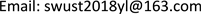Received: Mar. 4th, 2019; accepted: Mar. 19th, 2019; published: Mar. 27th, 2019ABSTRACT

In this paper, the displacement field and strain field of biomaterial-wild pig teeth are analyzed by MATLAB’s 2D digital image correlation program Ncorr. Through the high-definition camera, the digital image of the wild boar teeth before being subjected to external force and subjected to a small external force was obtained. The displacement of the center points of the two sets of digital images was determined by Newton-Rapshon algorithm, and the displacement changes and stress strains of the wild boar teeth under the action of tiny external forces were analyzed. Finally, through the analysis of the numerical calculation results, the displacement and stress changes at some specific positions are given, which provides a theoretical basis for the study of wild boar teeth.

Keywords:Wild Boar Tooth, Newton-Rapshon Algorithm, Strain Analysis

1西南科技大学理学院，四川 绵阳

2西南科技大学计算机科学与技术学院，四川 绵阳Copyright © 2019 by authors and Hans Publishers Inc.1. 引言

2. 数字图像的基本原理

2.1. 形函数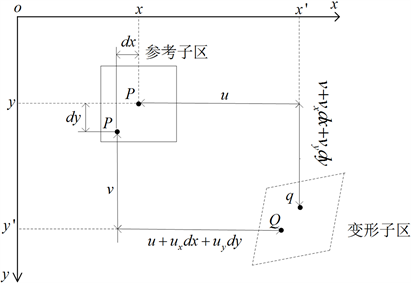Figure 1. Relation diagram of reference sub-region and deformation sub-region

$\left\{\begin{array}{l}{x}^{\prime }=x+u+\frac{\partial u}{\partial x}\Delta x+\frac{\partial u}{\partial y}\Delta y\\ {y}^{\prime }=y+v+\frac{\partial v}{\partial x}\Delta x+\frac{\partial v}{\partial y}\Delta y\end{array}$ (1)

$\left[\begin{array}{c}{x}^{\prime }\\ {y}^{\prime }\end{array}\right]=\left[\begin{array}{ccc}\begin{array}{c}1+{u}_{x}\\ {v}_{x}\end{array}& \begin{array}{c}{u}_{y}\\ 1+{v}_{y}\end{array}& \begin{array}{c}u\\ v\end{array}\end{array}\right]\left[\begin{array}{c}x\\ y\\ 1\end{array}\right]$ (2)

2.2. 相关准则

${C}_{f,g}\left(P\right)=\underset{x=-M}{\overset{M}{\sum }}\underset{y=-M}{\overset{M}{\sum }}{\left[\frac{f\left(x,y\right)}{\sqrt{\underset{x=-M}{\overset{M}{\sum }}\underset{y=-M}{\overset{M}{\sum }}\left[{f\left(x,y\right)\right]}^{2}}}-\frac{g\left({x}^{\prime },{y}^{\prime }\right)}{\sqrt{\underset{x=-M}{\overset{M}{\sum }}\underset{y=-M}{\overset{M}{\sum }}{\left[g\left({x}^{\prime },{y}^{\prime }\right)\right]}^{2}}}\right]}^{2}$ (3)

3. 试验测量与分析

3.1. 数字图像测量系统和试验材料介绍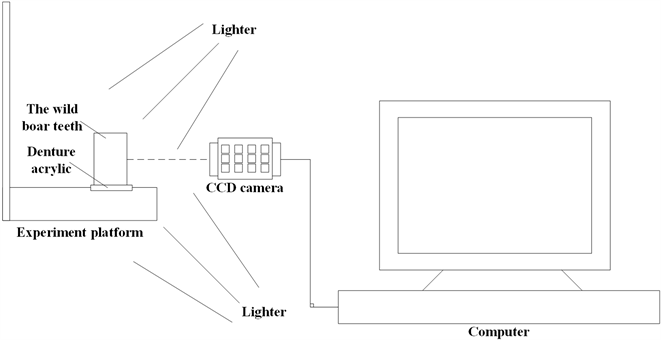Figure 2. Digital image experiment platform

3.2. 实验具体过程

1) 人工散斑：在实验开始前需要对样本喷洒人工散斑，具体做法为在试样表面喷上一薄层白色油漆作为底色，然后再喷上黑色油漆，从而得到黑白人工散斑。

2) 固定样本：实验中矩形框，即野猪牙齿，固定端四点固定在卷交机上，距离平台前端40 mm，并在矩形梁自由端施加1 kg的砝码，同时采集图像。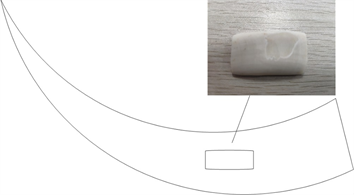Figure 3. Schematic diagram of wild boar's fangs

3) 实验参数设置：利用Ncorr软件对位移场和应变场进行分析。利用Ncorr软件计算应变场时，从参考图像中选择一个子集，并在变形图像中跟踪其相应位置。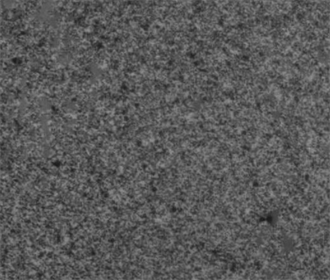Figure 4. Image before loading (reference image)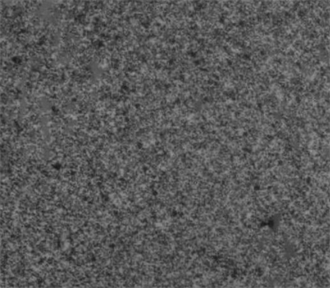Figure 5. Image after loading (target image)

3.3. 测量结果与分析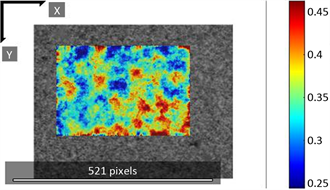Figure 6. Lateral displacement of material under external force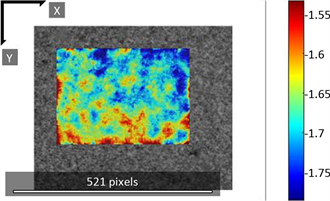Figure 7. Longitudinal displacement of material under external forces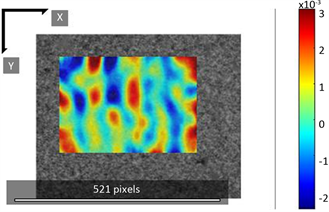Figure 8. Transverse stress variation of material under external force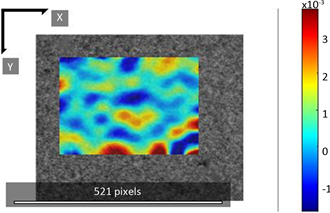Figure 9. Longitudinal stress change of material under external force

4. 结论Figure 10. Variation of lateral displacement in different regionsFigure 11. Variation of longitudinal displacement in different regions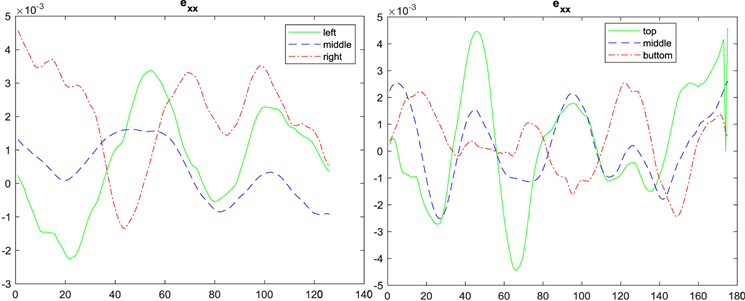Figure 12. Exx strain in different measuring areas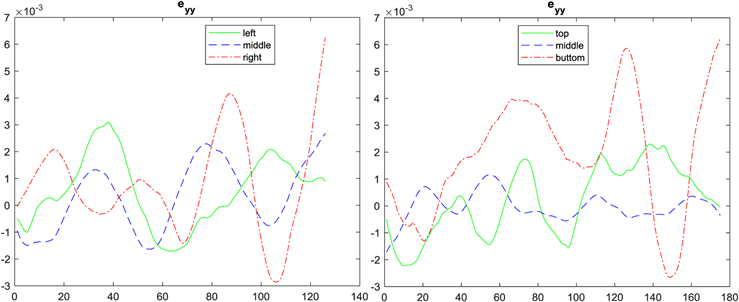Figure 13. Eyy strain in different measuring areas

Stress Analysis of Wild Boar Teeth under Tiny External Forces[J]. 力学研究, 2019, 08(01): 81-89. https://doi.org/10.12677/IJM.2019.81010

1. 1. Peter, W.H. and Ranson, W.F. (1982) Digital Imaging Techniques in Experimental Stress Analysis. Optical Engineering, 21, 427-431. https://doi.org/10.1117/12.7972925

2. 2. Corr, D. and Accardim Graham, B. (2007) Digital Image Correlation Analysis of Interfacial Debonding Properties and Fracture Behavior in Concrete. Engineering Fracture Mechanics, 74, 109-121. https://doi.org/10.1016/j.engfracmech.2006.01.035

3. 3. Desaic, K., Basu, S. and Parameswa, R. (2012) Determination of Complex Stress Intensity Factor for a Crack in a Bimaterial Interface Using Digital Image Correlation. Optics & Lasers in Engineering, 50, 1423-1430. https://doi.org/10.1016/j.optlaseng.2012.05.003

4. 4. Knauss, W.G., Chasiotis, I. and Huang, Y. (2003) Me-chanical Measurements at the Micron and Nanometer Scales. Mechanics of Materials, 35, 217-231. https://doi.org/10.1016/S0167-6636(02)00271-5

5. 5. Chasiotis, I. and Knauss, W.G. (2002) A New Microtensile Tester for the Study of MEMS Materials with the Aid of Atomic Force Microscopy. Experimental Mechanics, 42, 51-57. https://doi.org/10.1007/BF02411051

6. 6. 潘兵, 谢惠民, 续伯钦, 等. 应用数字图像相关方法测量含缺陷试样的全场变形[J]. 实验力学, 2007, 22(3-4): 379-384.

7. 7. Zhang, D.S. and Arola, D.D. (2004) Applications Tissues of Digital Image Correlation to Biological. Journal of Biomedical Optics, 9, 691-699. https://doi.org/10.1117/1.1753270

8. 8. Pan, B., Wang, Z. and Lu, Z. (2010) Genuine Full-Field Deformation Meas-urement of an Object with Complex Shape Using Reliability-Guided Digital Image Correlation. Optics Express, 18, 1011-1023. https://doi.org/10.1364/OE.18.001011

9. 9. Pan, B. (2009) Reliability-Guided Digital Image Correla-tion for Image Deformation Measurement. Applied Optics, 48, 1535-1542.

10. 10. Blaber, J., Adair, B. and Antoniou, E. (2015) Ncorr: Open-Source 2D Digital Image Correlation Matlab Software. Experimental Mechanics, 55, 1105-1122. https://doi.org/10.1007/s11340-015-0009-1

11. 11. Harilal, R. and Ramji, M. (2014) Adaptation of Open Source 2D DIC Software Ncorr for Solid Mechanics Applications. The 9th International Symposium on Advanced Science and Technology in Experimental Mechanics, New Delhi, India, 2014.

12. 12. 潘兵, 谢惠民, 戴福隆. 数字图像相关中亚像素位移测量算法的研究[J]. 力学学报, 2007, 39(2): 245-252.